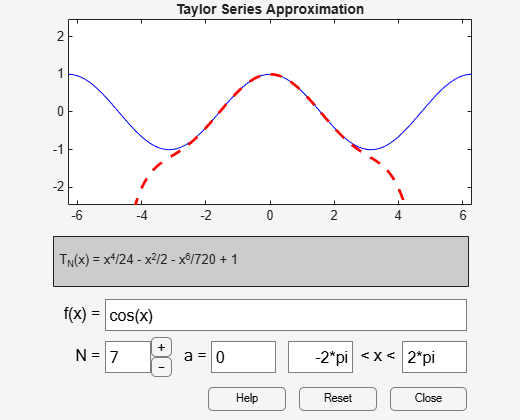# taylortool

Taylor series calculator

## Syntax

``taylortool``
``taylortool(f)``
``t = taylortool(___)``

## Description

example

````taylortool` initiates a GUI that computes the Taylor series expansion. The GUI that graphs a function against the Nth partial sum of its Taylor series about a base point `x = a`. The default function, value of N, base point, and interval of computation for `taylortool` are ```f = x*cos(x)```, `N = 7`, `a = 0`, and `[-2*pi,2*pi]`, respectively.```
````taylortool(f)` initiates the GUI that computes the Taylor series expansion of the given expression `f`.```

example

````t = taylortool(___)` returns the `Figure` object. Use `t` to query or modify properties of the figure after it is created.```

## Examples

collapse all

Open a GUI that computes the Taylor series expansion. By default, the GUI shows the Taylor series expansion of the function $\mathit{f}\left(\mathit{x}\right)=\mathit{x}\text{\hspace{0.17em}}\mathrm{cos}\left(\mathit{x}\right)$. The blue solid line shows the function $\mathit{f}\left(\mathit{x}\right)$ and the red dashed line shows its Taylor approximation.

`taylortool`Next, find the Taylor series expansion of the function $\mathrm{cos}\left(\mathit{x}\right)$. Assign an output variable to `taylortool` to return the `Figure` object.

```syms x t = taylortool(cos(x))``````t = Figure (Taylor Tool) with properties: Number: [] Name: 'Taylor Tool' Color: [0.9400 0.9400 0.9400] Position: [108 72 374.4000 302.4000] Units: 'points' Show all properties ```

Close the figure.

`close(t)`

## Input Arguments

collapse all

Input to approximate, specified as a symbolic expression or function.

## Version History

Introduced before R2006a